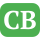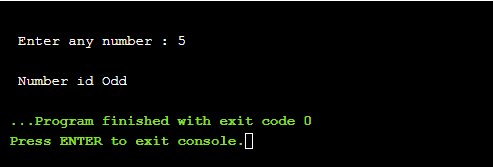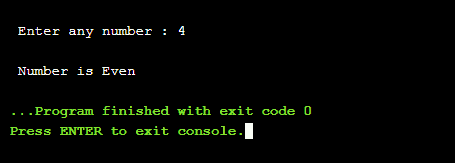CppBuzz.com

# C++ program to check if any number is even or odd

An even number is a number which has remainder of 0 upon division by 2, while an odd number is a number which has remainder of 1 upon division by 2. This C++ program uses modulas operator (%) to find if any number is even or odd.

``````#include#include

int main(){

int no;
std::cout<<"\n Enter any number : ";
std::cin>>no;

if(0 == no%2)
std::cout<<"\n Number is Even";
else
std::cout<<"\n Number id Odd";

return 0;
}
``````

## 5 is a odd number## 4 is a even number